# Material Analysis using Python

• Last Updated : 29 Sep, 2021

Using basic principles of more than one field to solve a complicated problem might be very difficult to reach by using one area of knowledge. With this kind of approach, one can also redefine problems outside usual boundaries and reach solutions using a new understanding of impenetrable situations which early considered impossible to get.

## Material Analysis

In layman’s terms, it is a field of study to analyze materials and their properties.

Scientifically-It’s a study to get insight into a material’s fundamental properties to determine whether the material is suitable for its intended use-case or needs some doping (or any other approach) to make it well qualified for the purpose.

Use-case: This study is also getting integrated with computer science to get better and precise insights into data without having much practical implementation.

Example: If one has a database of, say, Mn(Manganese) compounds and their magnetic behavior. Analyzing the same data can predict the magnetic properties of unknown magnetic compounds( whose properties are still novel) using a machine learning approach.

## Descriptor

In the dictionary, it is described as a word or expression used to describe or identify something.

A descriptor is used to describe the compound to computing algorithms. Many representations of properties elements can be converted in a mathematical format of vectors and matrices (like using one-hot vector encoding for describing an electronic configuration of an element ) to pass them as an input to a machine learning algorithm.

## Pymatgen Module

Pymatgen is a short form for Python Materials Genomics. It is a robust, open-source, and widely used Python library for material analysis.

Note- Only getting electronic configuration, atomic number, or any other very basic material properties does not account for material analysis.

Pymatgen is widely preferred as it is:

1. Highly flexible classes for representation of Element, Site, Molecule, Structure objects, Nearest Neighbors.
2. Variety of input/output formats like CIF, Gaussian, XYZ, VASP.
3. Electronic structure analyses, such as the density of states and band structure.
4. Powerful analysis tools.
5. Integration with Materials Project REST API, Crystallography Open Database, and other external data sources.
6. It is free to use, well documented, open and fast.

### Installation

As it is not an inbuilt python library so need to install it externally.

#### First Method:

The most straightforward installation is using conda. After installing conda:

conda install –channel conda-forge pymatgen

Pymatgen uses ‘gcc’ for compilation so the latest version of the same is required to compile pymatgen.

conda install gcc

#### Second Method:

Using pip:

pip install pymatgen

#### Third Method:

To install pymatgen on google collab

!pip install pymatgen

## Implementation

#### Details of an element and a compound

Fetching details(like atomic mass, melting point ) of an element using Element class of Pymatgen library. Pass the element symbol as a parameter to the Element class.

Similarly, can also get details of a compound.

## Python3

 `import` `pymatgen.core as pg` `# Fetch details of an Element` `fe ``=` `pg.Element(``"Fe"``)` `# Atomic mass` `print``(``'atomic mass: '``, fe.atomic_mass)` `print``(``'atomic mass: '``, fe.Z)` `# Melting point` `print``(``'melting point: '``, fe.melting_point)`   `# Fetch details of a composition` `cmps ``=` `pg.Composition(``"NaCl"``)` `print``(``'weight of composition: '``, cmps.weight)`   `# Composition allows strings to ` `# be treated as an Element object` `# It returns the number of Cl ` `# atoms present in the composition` `cmps[``"Cl"``]`

Output: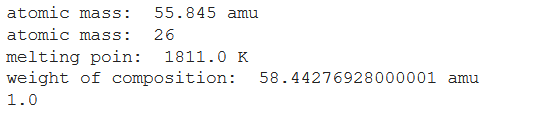#### Structure & file formats

Pymatgen has many libraries that are grouped/separated according to the properties they represent. Here, the pymatgen first diagonal lattice matrix is created followed by fetching its structure. Without a filename, a string is returned. Otherwise, the output is written to the file. If only the filename is provided

## Python3

 `# import module` `import` `pymatgen.core as pg` `from` `pymatgen.symmetry.analyzer ``import` `SpacegroupAnalyzer`   `# assign and display data` `lattice ``=` `pg.Lattice.cubic(``4.2``)` `print``(``'LATTICE\n'``, lattice, ``'\n'``)` `structure ``=` `pg.Structure(lattice, [``"Li"``, ``"Cl"``], ` `                         ``[[``0``, ``0``, ``0``], ` `                          ``[``0.5``, ``0.5``, ``0.5``]])` `print``(``'STRUCTURE'``, ``'\n'``, structure)`   `# Convert structure of the compound ` `# to user defined formats` `structure.to(fmt``=``"poscar"``)` `structure.to(filename``=``"POSCAR"``)` `structure.to(filename``=``"CsCl.cif"``)`

Output: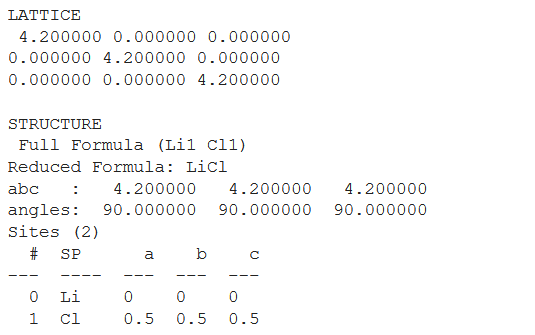#### Fetch Structure

Pymatgen also allows the user to read a structure from an external file. One can achieve the same in two ways using string and file that are used in the following code. The file we are going to fetch is the computed version of MnO2.cif.

## Python3

 `# Reading a structure from a file` `structure ``=` `pg.Structure.from_str(``open``(``"MnO2.cif"``).read(), ` `                                  ``fmt``=``"cif"``)` `structure ``=` `pg.Structure.from_file(``"MnO2.cif"``)`   `# Reading a molecule from a file` `graphite ``=` `pg.Molecule.from_file(``"graphite.xyz"``)` `# Writing the same molecule but in other file format` `mol.to(``"graphite.cif"``)`

Output: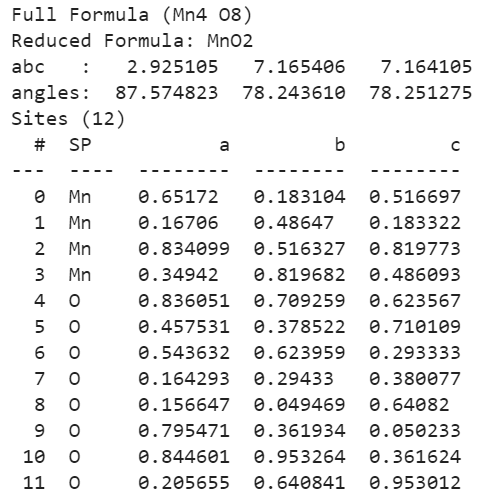It can also work as a file converter, as it allows to read a molecule from a file in one format and write the same molecule in a file of another format.

#### External Data Sources

As explained above that pymatgen can be linked to different external data sources. Material Project’s data can be accessed in pymatgen using the MPRester API of the project.

The Materials Project is one of those external databases that make available its data and scientific analysis through the open Materials Application Programming Interface API (also known as MPRester API as it is based on REpresentational State Transfer (REST) principles). This API can conceivably be used with any programming language supporting basic HTTP requests, a wrapper to MPRester API has already been implemented in pymatgen library to facilitate researchers who want to utilize their data.

Refer to this website for API key generation -> https://materialsproject.org/open

Here first, the object is created of the API key followed by querying the properties’ data of a particular task id( task id can be thought of as a unique identity of each element present in the database of Material Project).

Note- property names are mentioned under properties. If no such particular property data present then a null object is received for that particular property.

## Python3

 `# import module` `from` `pymatgen.ext.matproj ``import` `MPRester`   `# create object` `m ``=` `MPRester(API_key)` `# fetch all the required properties of an element using mpid` `# fetching details of a compound related to TaskId=mpid-1010` `data_one ``=` `m.query(criteria``=``{``'task_id'``: ``'mp-1010'``},` `                   ``properties``=``[``"full_formula"``, ` `                               ``"spacegroup.symbol"``, ` `                               ``"volume"``,` `                               ``"nsites"``, ``"density"``, ` `                               ``"spacegroup.crystal_system"``,` `                               ``"final_energy_per_atom"``, ` `                               ``"final_energy"``])`   `# display fetched data` `print``(data_one)`

The output format is in the dictionary data structure for easy and understandable access to the required property

Output: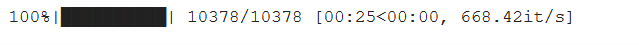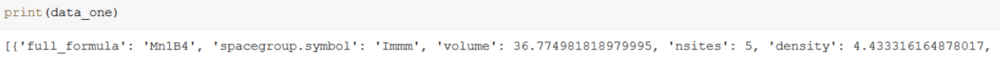Secondly, fetching all the defined properties data (elements and compounds) of iron(Fe) compounds.

## Python3

 `# Fetch all the compounds details of an element in the database` `# Fetching data of Fe-Iron` `from` `pymatgen.ext.matproj ``import` `MPRester`   `import` `pandas as pd` `m ``=` `MPRester(API_key)` `data_s ``=` `m.query(criteria``=``{``"elements"``: {``"\$in"``: [``"Fe"``]}},` `                 ``properties``=``[``"full_formula"``, ` `                             ``"spacegroup.symbol"``, ` `                             ``"volume"``,` `                             ``"nsites"``, ``"density"``, ` `                             ``"spacegroup.crystal_system"``,` `                             ``"final_energy_per_atom"``, ` `                             ``"final_energy"``])`   `# convert data to pandas data ` `# frame and store it in .csv file` `df ``=` `pd.DataFrame(data_s)` `df.to_csv(``'all.csv'``)`   `# display data saved in all.csv` `print``(df)`

The output of querying all data of Fe element is in nested dictionary format and is very large to show in the console so it is first converted to a pandas data frame followed by saving it as a .csv file.

OUTPUT: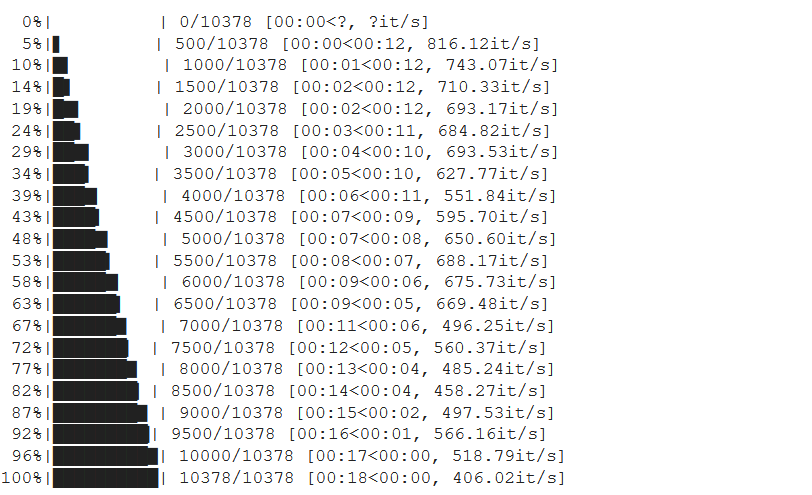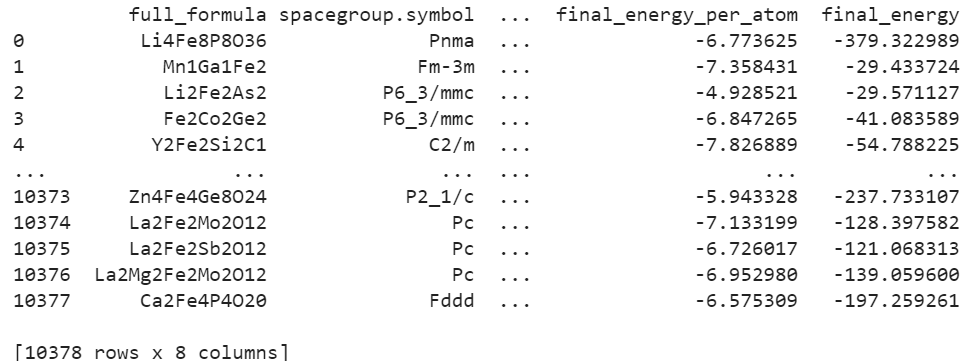## Real-Life Use case

Here, we are going to count the number of atoms in a compound. It can easily be done by fetching structural details of the compound in CIF format as it contains all the coordinate locations of each and every atom of the compound.

First, remove all the unnecessary text from the file and then count the number of remaining lines.

CoNi3 compound is associated with mp-1183751

## Python3

 `# import module` `from` `pymatgen.core ``import` `Structure` `from` `pymatgen.ext.matproj ``import` `MPRester` `import` `re`   `m ``=` `MPRester(API_key)` `id` `=` `'mp-1183751'` `data_c ``=` `m.query(criteria``=``{``'task_id'``: ``id``}, ` `                 ``properties``=``[` `                 ``'cifs.conventional_standard'``])`   `# delete extras` `with ``open``(``'cnt.cif'``, ``'w'``) as f:` `    ``filedata ``=` `str``(data_c)` `    ``filedata ``=` `re.sub(r``'.*_occupancy'``, ` `                      ``'', filedata)` `    ``filedata ``=` `filedata[:``-``4``]` `    ``filedata ``=` `filedata.replace(``'\\n'``, ` `                                ``'\n'``)` `    ``f.write(filedata[``1``:])` `    ``f.close()` `    ``count ``=` `len``(``open``(``'cnt.cif'``, ` `                     ``'r'``).readlines())` `    `  `    ``# display the no. of atoms` `    ``print``(count)`

Output:

`4`

My Personal Notes arrow_drop_up
Recommended Articles
Page :